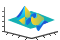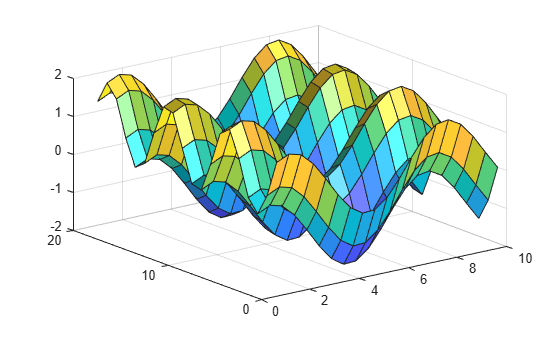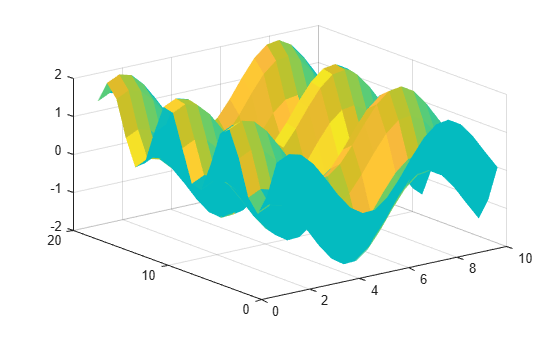surfl

Surface plot with colormap-based lighting

•Description

example

surfl(X,Y,Z) creates a three-dimensional surface plot with highlights from a light source. The function plots the values in matrix Z as heights above a grid in the x-y plane defined by X and Y. The function uses the default direction for the light source and the default lighting coefficients for the shading model. This sets the color data for the surface to be the reflectance of the surface.

Because of the way surface-normal vectors are computed, surfl requires matrices that are at least 3-by-3.

surfl(Z) creates a surface and uses the column and row indices of the elements in Z as the x- and y-coordinates.

example

surfl(___,'light') creates a surface with highlights from a MATLAB® light object. This produces different results from the default colormap-based lighting method. Specify the 'light' object as the last input argument.

example

surfl(___,s) additionally specifies the direction of the light source.

example

surfl(X,Y,Z,s,k) additionally specifies the reflectance constant.

surfl(ax,___) plots into the axes specified by ax instead of the current axes. Specify the axes as the first input argument.

example

s = surfl(___) returns the chart surface object. If the light source is specified as a light object using the 'light' option, then s is returned as a graphics array that includes the chart surface object and the light object. Use s to modify the surface and light object after it is created. For a list of properties, see Surface Properties and Light Properties.

Examples

collapse all

Create three matrices of the same size. Then plot them as a surface using colormap-based lighting. The surface uses Z for height and both Z and the light source for color.

[X,Y] = meshgrid(1:0.5:10,1:20);
Z = sin(X) + cos(Y);
surfl(X,Y,Z)Create three matrices of the same size. Then plot them as a surface with highlights from a MATLAB® light object. The surface uses Z for height and both Z and the light object for color. The function returns an array containing a surface object and a lighting object. Assign it to the variable sl.

[X,Y] = meshgrid(1:0.5:10,1:20);
Z = sin(X) + cos(Y);
sl = surfl(X,Y,Z,'light');Index into sl to access and modify properties of the surface object and the light object after they are created. The surface plot is accessible as sl(1) and the light object as sl(2). For example, change the color of the light by setting the Color property of the light object.

sl(2).Color = 'r';Create three matrices of the same size to plot as a surface. Specify the direction of the light source to have an azimuth of 45 degrees and an elevation of 20 degrees. Increase the reflectance of the surface by increasing the contribution of ambient light and decreasing the contibutions of diffused and specular reflection. Assign the surface object to the variable sl.

[X,Y] = meshgrid(1:0.5:10,1:20);
Z = sin(X) + cos(Y);
s = [-45 20];
k = [.65 .4 .3 10];

Plot the data using the source and reflectance vectors.

sl = surfl(X,Y,Z,s,k);Use sl to access and modify properties of the surface object after it is created. For example, hide the edges by setting the EdgeColor property.

sl.EdgeColor = 'none';Input Arguments

collapse all

x-coordinates, specified as a matrix the same size as Z, or as a vector with length n, where [m,n] = size(Z). If you do not specify values for X and Y, surfl uses the vectors (1:n) and (1:m).

You can use the meshgrid function to create X and Y matrices.

The XData property of the Surface object stores the x-coordinates.

Example: X = 1:10

Example: X = [1 2 3; 1 2 3; 1 2 3]

Example: [X,Y] = meshgrid(-5:0.5:5)

Data Types: single | double | int8 | int16 | int32 | int64 | uint8 | uint16 | uint32 | uint64

y-coordinates, specified as a matrix the same size as Z or as a vector with length m, where [m,n] = size(Z). If you do not specify values for X and Y, surfl uses the vectors (1:n) and (1:m).

You can use the meshgrid function to create the X and Y matrices.

The YData property of the surface object stores the y-coordinates.

Example: Y = 1:10

Example: Y = [1 1 1; 2 2 2; 3 3 3]

Example: [X,Y] = meshgrid(-5:0.5:5)

Data Types: single | double | int8 | int16 | int32 | int64 | uint8 | uint16 | uint32 | uint64

z-coordinates, specified as a matrix. Z must have at least two rows and two columns.

The ZData property of the surface object stores the z-coordinates.

Example: Z = [1 2 3; 4 5 6]

Example: Z = sin(x) + cos(y)

Data Types: single | double | int8 | int16 | int32 | int64 | uint8 | uint16 | uint32 | uint64

Direction from the surface to the light source, specified as a two- or three-element vector. The vector has the form [sx sy sz] or [azimuth elevation]. The default direction is 45° counterclockwise from the current view direction.

Reflectance constant, specified as a four-element vector. The vector defines the relative contributions of ambient light, diffused reflection, specular reflection, and the specular shine coefficient using the form [ka kd ks shine]. By default, k is [.55 .6 .4 10].

Axes to plot in, specified as an axes object. If you do not specify the axes, then surfl plots into the current axes.

Tips

• The ordering of points in the X, Y, and Z matrices defines the inside and outside of parametric surfaces. To have the opposite side of the surface reflect the light source, use surfl(X',Y',Z').